# Does the N64 joystick really allow 360 degrees of control?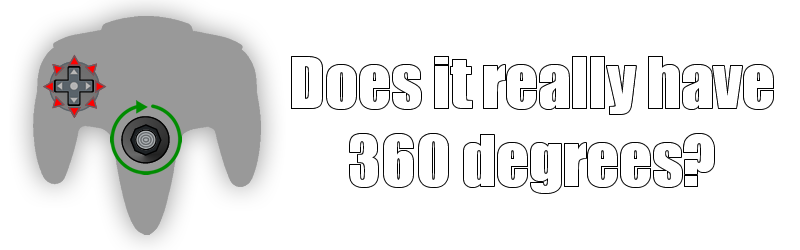One thing I keep on hearing time and time again is that the Nintendo 64 controller was revolutionary because the analogue joystick allows for 360 degrees of control compared to the 8 directions of the classical D-Pad. This is true, it meant for much smoother control in three dimensions and has been a standard for controllers ever since.

But then I got wondering… Does it really allow for 360 degrees, or is that just a figure of speech? Let’s find out.

## How the N64 controller reads input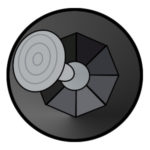The Nintendo 64 controller feeds the console a 32-bit integer which represents the current buttons pressed, along with the joystick’s inclination. The buttons occupy 16 of those bits (though two aren’t used since the N64 only has 14 buttons). The joystick input is represented by two 8-bit integers that show how much incline there is on the x-axis and y-axis.

Code definition
Here’s a bit of code that defines how the N64 operating system records controller input as a struct:

```typedef struct {
u16     button;
s8      stick_x;
s8      stick_y;
u8	errno;
Since these values are signed it means that in theory, the joystick can have a range from -128 through to 127.

The range of -128 to 127 is only in theory though; those are just upper and lower limits. According to the N64 developer documentation:

Control Stick resolution differs from Controller to Controller. Moreover, as a Controller gets old, the uppermost value that the Control Stick can input can decline. To handle the variation between Control Sticks, we recommend you set the actual usable range to the values shown below.

Left/right X axis +/- 61
Up/down Y axis +/- 63
X axis incline +/- 45
Y axis incline +/- 47

This means that when pushing the joystick left or right, the max value used in a program should be 61. Similarly, 63 should be the maximum value for the up and down.

Since the N64 controller joystick resembles an octagon, the maximum diagonal values approximate 70.7% of their maximum horizontal/vertical values.

## Actual joysticks max incline

Since there are dozens of joystick types, I decided to try out some real life joysticks with nu1 to find out what the maximum values were for each axis (not counting diagonals).

As you can see, the controller that best approximates the maximum values is the Retro fighters controller. The worn out official controller is the one that barely makes the cut for the minimum requirements. This is the reason why old controllers might not work properly; any more wear on the joystick and it can no longer reach the maximum value for X and Y.

This also comes to show that insensitive controllers like the worn out ones have most of their operational range towards the outer tilts of the controller, while better controllers achieve maximum tilt about 2/3rds of the way out.

## The calculation

First let’s do a calculation to find out all the possible angles which the N64 can process based on the maximum range of X and Y (-128 to +127).

The calculation is a simple trigonometry function, using the arc tangent function on the ratio of a specific X and Y coordinate to find the angle theta (θ).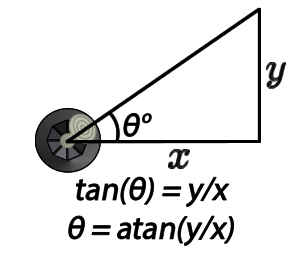Next, we need to repeat the calculation for every possible coordinate.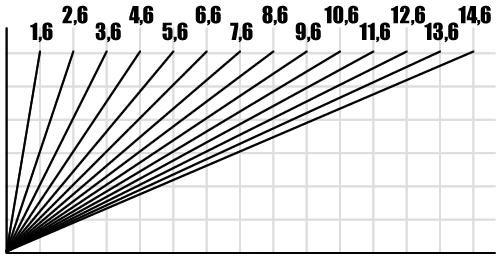There are some coordinates which will repeat themselves because they share the same ratio of X and Y. We’ll exclude them since that will cause duplicate angles on our list.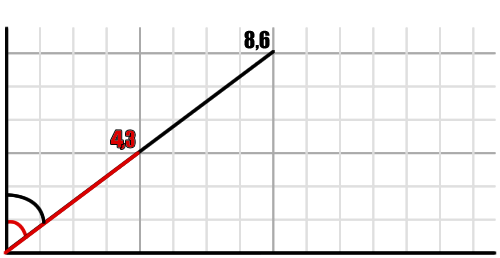It’s really as simple as that. Repeat the calculation for each X/Y value and remove duplicates. That’s all there is to it.

## The full grid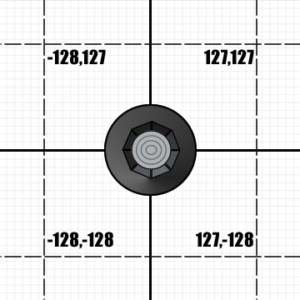The simplest calculation is to do the full grid, from -128 to +127. It will be a lot of values, but I’ll get a computer to do the job and save some time. Here’s the PHP script I’ll be using:

Expand for code
```<?php
\$minx = -128;
\$miny = -128;
\$maxx = 127;
\$maxy = 127;
\$values = array();
for (\$x = \$minx; \$x <= \$maxx; \$x++){
for (\$y = \$miny; \$y <= \$maxy; \$y++){ \$val = \$x != 0 ? atan(\$y/\$x) : acos(2); if (is_nan(\$val)){ \$val = pi()/2*(\$y!=0?\$y/abs(\$y):1); } \$val = \$val/pi()*180; \$val += 90; \$val = \$x >= 0 ? \$val : \$val + 180;
if (!in_array(\$val,\$values)){
\$values[] = \$val;
}
}
}
asort(\$values);
\$total = 0;
foreach (\$values as \$v){
echo \$v."
";
\$total++;
}
echo "Total: \$total.";```

It’s fairly straight forward. The script scans through every possible coordinate and calculates the joystick’s angle. It also handles some exceptions, to avoid division by zero and the like.

The result of the script is amazingly big. There are a total of 39920 possible angles when using the full range of a controller’s input. Here’s a sample of the beginning and to give you an idea of what I’m talking about:

Angle sample
```0
0.44761417086055
0.45113854678728
0.45471886169314
0.45835645800042
0.46205272143077
0.46580908276499
0.46962701968964
0.47350805873492
0.47745377730958
0.48146580583834
0.48554583000814
0.48969559312923
0.49391689861875
0.49821161261363
0.50258166672101
0.50702906091477
0.51155586658705
0.51616422976484
0.52085637450195
0.52563460645761
0.53050131667381
0.53545898556453
0.54051018713068
0.54565759341571
0.55090397921856
0.55625222708066
0.56170533256655
0.56726640985794
0.57293869768347
0.57872556560775
0.58463052070518
0.59065721464667
0.59680945122918
0.60309119438053
0.60950657667519
0.61605990839924
0.62275568720632
0.62959860841026
0.63659357596349
0.64374571417538
0.65106038022948
0.6585431775636
0.66619997018314
0.67403689798451
0.68206039317265
0.69027719786507
0.69869438298348
0.70731936854426
0.71615994547041
0.72522429905925
0.73452103425481
0.74405920288869
0.75384833307076
0.76389846092999
0.77422016492805
0.78482460299189
0.79572355273926
0.80692945510236
0.81845546168861
0.83031548625802
0.8425242607404
0.85509739626671
0.86805144974554
0.88140399658214
0.89517371021107
0.90222116153015
0.90938044919913
0.91665425638529
0.92404535277271
0.93155659807748
0.93919094573558
0.94695144677347
0.95484125387217
0.96286362563622
0.97102193107916
0.97931965433948
0.98776039963981
0.99634789650491
1.0050860052542
1.0139787227853
1.0230301886678
1.0322446915657
1.04162667601
1.0511807495437
1.0609116902642
1.070824454787
1.0809241866607
1.0912162252626
1.1017061152064
1.1123996162978
1.1233027140754
1.134421630977
1.1457628381751
1.1573330681295
1.1691393279074
1.1811889133266
1.193489423982
1.20604877922
1.2188752351313
1.2319774026397
1.2453642667683
1.2590452071753
1.2730300200567
1.287328941525
1.3019526725789
1.3169124057933
1.3322198538696
1.3426240265378
1.3478872801986
1.3531919537797
1.3639275316029
1.3748347805694
1.3803540734444
1.3859178508122
1.3971810272964
1.4086287353385
1.4144232114022
1.4202655463991
1.4320961841646
1.4441255309358
1.4502162909334
1.4563586343397
1.4688007143858
1.4814571708871
1.4878675288278
1.4943335912665
1.507435758775
1.5207696611438
1.5275254422129
1.5343414997008
1.548157698978
1.5622249168424
1.569354804749
1.5765500551837
1.5911402711946
1.6060029892822
1.6135389328812
1.6211459136534
1.6365770416167
1.6523046776513
1.6602823689828
1.6683374482933
1.6846843178963
1.7013546053261
1.7098140441415
1.7183580016555
1.7357045889284
1.7534048600715
1.7623910236605
1.7714697400341
1.7899106082461
1.8039951162006
1.8087393224921
1.8183029644518
1.827968244305
1.832839505942
1.8476102659946
1.8626209493067
1.8676788394342
1.8778774472854
1.8881880067409
1.8933858457463
1.9091524329964
1.9251837083231
1.930587441167
1.9414863909144
1.9525090493996
1.958067429234
1.974934010882
1.9920935729664
1.9978798564767
2.0095538130211
2.021364940356

...

358.00212014352
358.00790642703
358.02506598912
358.04193257077
358.0474909506
358.05851360909
358.06941255883
358.07481629168
358.090847567
358.10661415425
358.11181199326
358.12212255271
358.13232116057
358.13737905069
358.15238973401
358.16716049406
358.1720317557
358.18169703555
358.19126067751
358.1960048838
358.21008939175
358.22853025997
358.23760897634
358.24659513993
358.26429541107
358.28164199834
358.29018595586
358.29864539467
358.3153156821
358.33166255171
358.33971763102
358.34769532235
358.36342295838
358.37885408635
358.38646106712
358.39399701072
358.40885972881
358.42344994482
358.43064519525
358.43777508316
358.45184230102
358.4656585003
358.47247455779
358.47923033886
358.49256424123
358.50566640873
358.51213247117
358.51854282911
358.53119928561
358.54364136566
358.54978370907
358.55587446906
358.56790381584
358.5797344536
358.5855767886
358.59137126466
358.6028189727
358.61408214919
358.61964592656
358.62516521943
358.6360724684
358.64680804622
358.6521127198
358.65737597346
358.66778014613
358.68308759421
358.69804732742
358.71267105847
358.72696997994
358.74095479282
358.75463573323
358.76802259736
358.78112476487
358.79395122078
358.80651057602
358.81881108667
358.83086067209
358.84266693187
358.85423716182
358.86557836902
358.87669728592
358.8876003837
358.89829388479
358.90878377474
358.91907581334
358.92917554521
358.93908830974
358.94881925046
358.95837332399
358.96775530843
358.97696981133
358.98602127721
358.99491399475
359.0036521035
359.01223960036
359.02068034566
359.02897806892
359.03713637436
359.04515874613
359.05304855323
359.06080905426
359.06844340192
359.07595464723
359.08334574361
359.0906195508
359.09777883847
359.10482628979
359.11859600342
359.13194855025
359.14490260373
359.15747573926
359.16968451374
359.18154453831
359.1930705449
359.20427644726
359.21517539701
359.22577983507
359.23610153907
359.24615166693
359.25594079711
359.26547896575
359.27477570094
359.28384005453
359.29268063146
359.30130561702
359.30972280213
359.31793960683
359.32596310202
359.33380002982
359.34145682244
359.34893961977
359.35625428582
359.36340642404
359.37040139159
359.37724431279
359.3839400916
359.39049342332
359.39690880562
359.40319054877
359.40934278535
359.41536947929
359.42127443439
359.42706130232
359.43273359014
359.43829466743
359.44374777292
359.44909602078
359.45434240658
359.45948981287
359.46454101444
359.46949868333
359.47436539354
359.4791436255
359.48383577024
359.48844413341
359.49297093909
359.49741833328
359.50178838739
359.50608310138
359.51030440687
359.51445416999
359.51853419416
359.52254622269
359.52649194127
359.53037298031
359.53419091724
359.53794727857
359.541643542
359.54528113831
359.54886145321
359.55238582914```

So in this case, the N64 controller does technically have input for more than 360 degrees. In fact, it averages out to over 100 unique results for each available degree.

## Adapting the calculation for expected input

Since the Nintendo 64 controller joystick isn’t perfect, games have to put practical limits on the maximum X and Y values, as mentioned before. This would result in a limit diagram that looks something like this: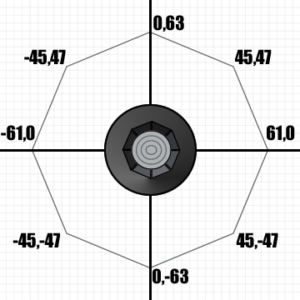This means that we’re going to have to get a bit more creative with our calculation, given that it has an octagon shape. In order to do this, we’re going to need to do two things:

1. Reduce the range from -61 to +61 on the x-axis and -63 to+63 on the y-axis
2. Make it so that only values inside the hexagon are counted.

The first bit is easy, since it only involves changing the initial values. Simply changing the min/max values makes the total angles go down to 9556, or about 25 angles per degree.

The second part quires a little bit of ingenuity. We need to consider the octagon as a series of eight lines, each of which has a formula. Four of them appear above the x-axis, and another four appear under the x-axis. For the ones above, we want to find points whose y-value is under the line, and vice versa for those under the x-axis. I think it’s a bit more clear if you see it in an image: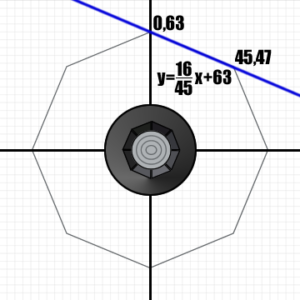The values in this case have to be below the blue line. If we repeat the calculation for the eight lines, it should tell us whether the point we’re calculating for falls within the octagon. Here are all the equations, for reference (click to enlarge):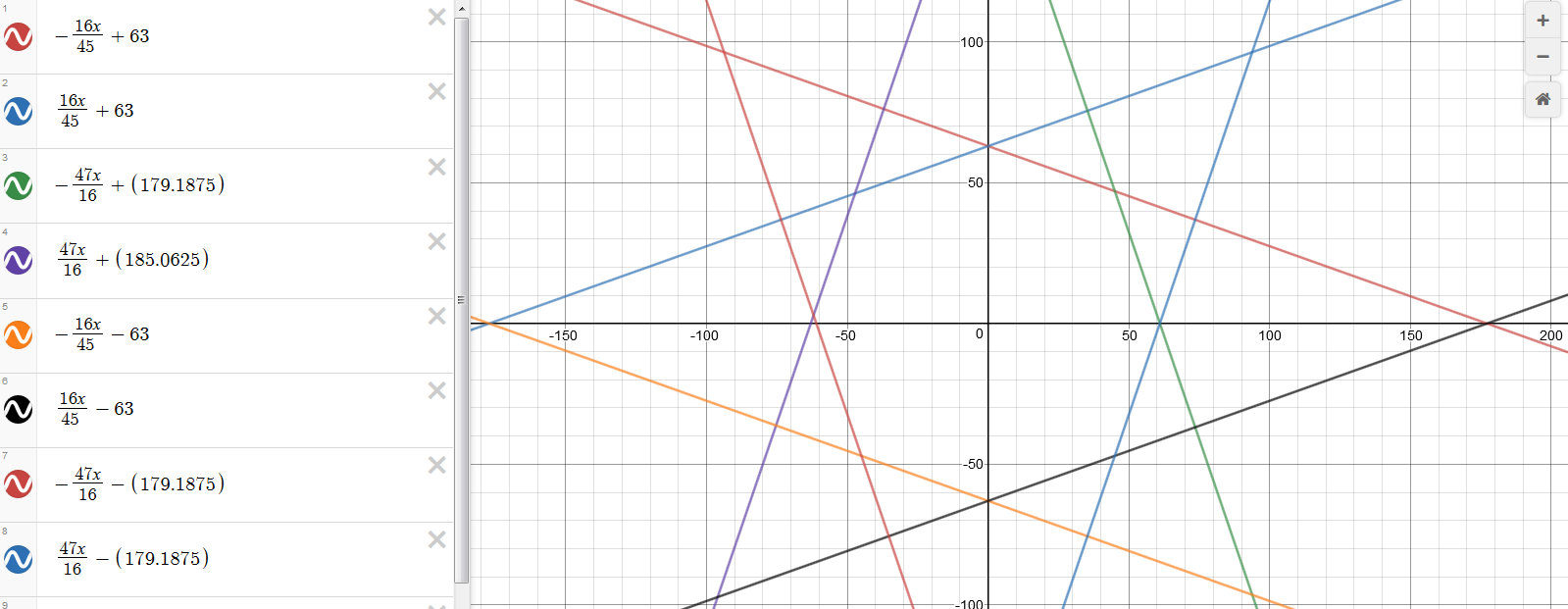Let’s use these equations to add a while bunch of ifs to the code. It’ll make things a lot dirtier, but it’ll make sense once you see it.

Expand for code
```for (\$x = \$minx; \$x <= \$maxx; \$x++){
for (\$y = \$miny; \$y <= \$maxy; \$y++){
if(\$y < (\$x*16/45)+63) {
if(\$y < (\$x*-16/45)+63) {
if(\$y < (\$x*-47/16)+179.1875) {
if(\$y < (\$x*-47/16)+179.1875) { if(\$y > (\$x*16/45)-63) {
if(\$y > (\$x*-16/45)-63) {
if(\$y > (\$x*-47/16)-179.1875) {
if(\$y > (\$x*-47/16)-179.1875) {```

When we run the script we end up with a smaller amount of 7380 possible angles, a whole 2176 less than before. This means that each ‘corner’ we chapped off contained 544 unique angles associated to it.

## Conclusion: are there more than 360 degrees?

So after doing all those calculations, we ended up with three values:

• 39920 possible angles if you consider the full range of values for -127 to +128. These are for all the possible inputs considering the limitations of the controller input format.
• 9556 possible angles for the full range for ±61x and ±63y. Since controllers don’t allow for input all the way to -128/127, these are limitations recommended by the documentation to allow even old worn out controllers to work.
• 7680 possible angles when you take into consideration the octagonal shape of the Nintendo 64 controller.

Even under the most restrictive conditions, you can tell that there are a lot more than 360 degrees. In fact, there are about 21.33 angles per degree.

The only question left is to know whether all of these can be used by the N64 as floating point numbers. I suppose that the only thing that might change is the accuracy of each angle, but since they only really need to be accurate to 5 decimals or so to be unique, it should hold up just fine. If anything, that’s an effort for another day.

You can find files detailing all the individual angles that were calculated here.

### Articles across the web

I keep on hearing how the Nintendo 64 controller allows the player to have 360 degrees of control during gameplay. How true is this really?
Article published on N64 Squid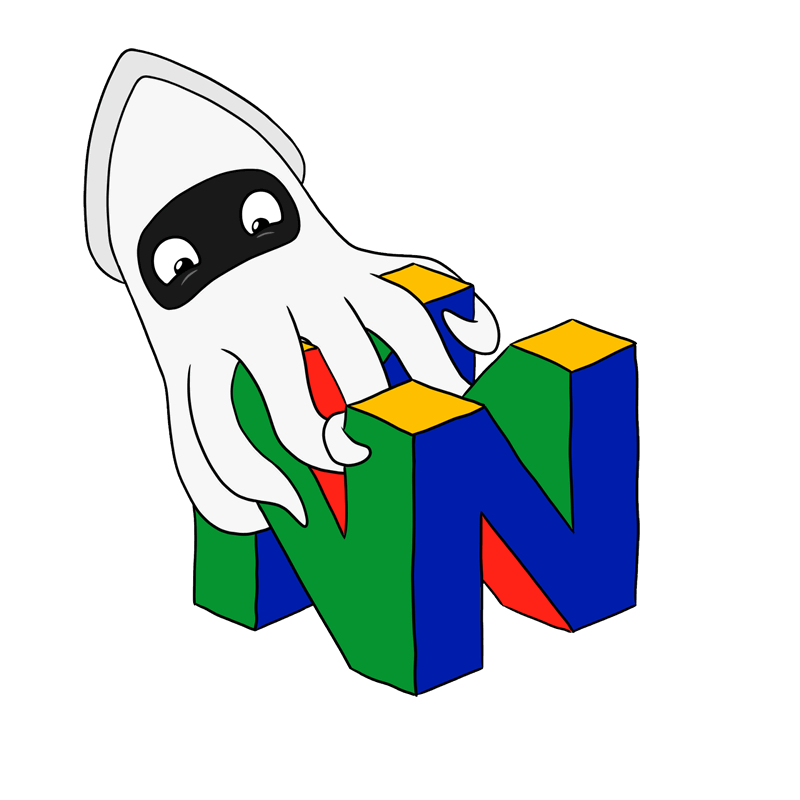## 2 COMMENT

•### Rodolfo

Thank you so much for this! I find this post very interesting and instructive for people that wonders about the influence of the octogonal shape in the number of angles per degree

•